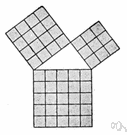# theorem

(redirected from theorematical)
Also found in: Thesaurus, Medical, Encyclopedia.

## the·o·rem

(thē′ər-əm, thîr′əm)
n.
1. An idea that has been demonstrated as true or is assumed to be so demonstrable.
2. Mathematics A proposition that has been or is to be proved on the basis of explicit assumptions.

[Late Latin theōrēma, from Greek, from theōrein, to look at, from theōros, spectator; see theory.]

## theorem

(ˈθɪərəm)
n
(Logic) maths logic a statement or formula that can be deduced from the axioms of a formal system by means of its rules of inference
[C16: from Late Latin theōrēma, from Greek: something to be viewed, from theōrein to view]

## the•o•rem

(ˈθi ər əm, ˈθɪər əm)

n.
1. Math. a theoretical proposition, statement, or formula embodying something to be proved from other propositions or formulas.
2. a rule or law, esp. one expressed by an equation or formula.
3. Logic. a proposition that can be deduced from the premises or assumptions of a system.
4. an idea, method, or statement generally accepted as true or worthwhile without proof.
[1545–55; < Late Latin theōrēma < Greek theṓrēma spectacle, object of contemplation, theorem =theōrē-, variant s. of theōreîn to observe, derivative of theōrós person sent to consult an oracle, spectator + -ma resultative n. suffix]

## the·o·rem

(thē′ər-əm, thîr′əm)
A mathematical statement whose truth can be proved on the basis of a given set of axioms or assumptions.
ThesaurusAntonymsRelated WordsSynonymsLegend:
 Noun 1theorem - a proposition deducible from basic postulatesbinomial theorem - a theorem giving the expansion of a binomial raised to a given powerproposition - (logic) a statement that affirms or denies something and is either true or false 2 theorem - an idea accepted as a demonstrable truthidea, thought - the content of cognition; the main thing you are thinking about; "it was not a good idea"; "the thought never entered my mind"Bayes' theorem - (statistics) a theorem describing how the conditional probability of a set of possible causes for a given observed event can be computed from knowledge of the probability of each cause and the conditional probability of the outcome of each cause

## theorem

noun He postulated a theorem and proved it.

## theorem

noun
A broad and basic rule or truth:
Translations
نَظَرِيَّه
teorém
læresætning
elméleti tétel
setning
teorema
teorēma
teoréma
davateorem

## theorem

[ˈθɪərəm] N (Math) →

[ˈθiːərəm] n

## theorem

nSatz m (also Math), → Theorem nt (geh, spec)

## theorem

[ˈθɪərəm] n (Math) →

## theorem

(ˈθiərəm) noun
especially in mathematics, something that has been or must be proved to be true by careful reasoning. a geometrical theorem.
Site: Follow: Share:
Open / Close